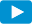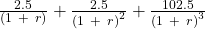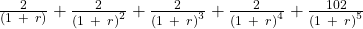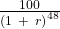Notes for Level I CFA® Program

R52 Introduction to Fixed Income Valuation

2.2. Yield-to-MaturityWatch VideoThe yield-to-maturity (YTM) is the internal rate of return on the cash flows – the uniform interest rate that will make the sum of the present values of future cash flows equal to the price of the bond. It is the implied market discount rate. In simpler terms, it is a bond’s internal rate of return – the rate of return on a bond including interest payments and capital gain if the bond is held until maturity. Yield-to-maturity is based on three important assumptions:

• The investor holds the bond to maturity.
• The issuer does not default on payments and pays coupon and principal as they come due.
• The investor is able to reinvest all proceeds (coupons) at the YTM. (This is an unrealistic assumption as the interest rates may increase or decrease after the bond is purchased. So the coupon may be reinvested at a higher or lower rate.)

Example

A $100 face value bond with a coupon rate of 10% has a maturity of 4 years. The price of the bond is$80. What is its yield-to-maturity?

Solution:

Based on YTM’s definition, we come up with the equation below:

80 =This equation can be solved using trial and error but it is much easier to use a financial calculator: N = 4; PMT = 10; FV = 100; PV= -80; CPT I/Y = 17.34%. The bond is trading at a discount. So the coupon rate (10%) must be lower than market rate (17.34%).

Example

Calculate the yields-to-maturity for the following bonds:

 Bond Coupon Payment per Period Number of Periods to Maturity Price A 2.50 3 102.80 B 2.00 5 97.76 C 0.00 48 23.425

Solution:

Bond A: 102.80 =, r = 1.54%
Bond B: 97.76 =, r = 2.48%
Bond C: 23.425 =, r = 3.07%